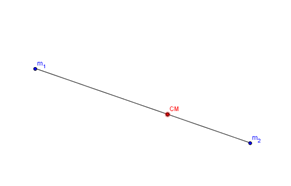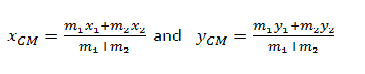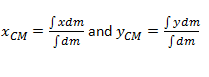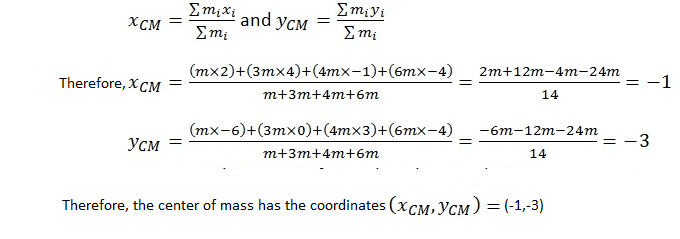# How to Find the Center of Mass

## Center of Mass – Definition

The point at which the whole mass of a body or system may be considered to be concentrated is known as the center of mass. In other words, it is the point where the total mass of the body or the system has the same effect when concentrated to a point mass.

## Calculating Center of Mass

A rigid body has a continuous mass distribution. A system of masses may have either continuous or discrete mass distribution. To understand the concept better let’s consider a system of two point masses mand m2 positioned at (x1,y1)and (x2,y2).The center of mass of the system will be given by the coordinates (xCM,yCM) obtained by the following formula.If the z coordinates are also given then z coordinates of the center of mass can be obtained by the same method. The center of mass internally divides the distance between the two points and the distance from CM to each mass (r) isinversely proportional to the mass(m). i.e. r∝1/m. Therefore, following relation holds for any two point mass systems. r1/r2 =m2/m1. The result for two point masses can be extended to many particle systems as follows.If the coordinates of the particle mi are given by (xi,yi ) then the coordinates of the center of mass of the many particle system is given by,

A continuous mass distribution can be approximated as a collection of infinitesimal masses. Therefore, taking the limiting cases of the above results provides the coordinates of the center of mass.If the object has uniform mass distribution (uniform density) and regular geometric object, the center of mass lies at the geometric center of the object. It should also be noted that, center of mass (CM) and center of gravity (CG) are used synonymously in most situations. However, they are different, and they coincide only when the gravitational field acting upon the body or system is uniform. Otherwise, the center of mass and center of gravity are separated.

This is true for all the objects in the earth`s gravitational field. However, the difference in the locations of the center of mass and center of gravity is too small for small objects, but for large objects, especially tall objects such as a rocket on its launch pad, there is a significant separation between the center of mass and center of gravity.

## How to Find the Center of Mass – Example

Center of Mass Example 01. Masses m,3m,4m and 6m are located at coordinates (2,-6),(4,0),(-1,3) and (-4,-4) respectively. Find the center of mass of the system.Center of Mass Example 02. Moon orbits at 385000 km away from the center of the earth. If the mass of the moon is 7.3477 × 1022 kg or 0.012300 of Earth’s mass, find the distance to the center of mass of earth and moon system, from earth’s center.

From the relation r1/r2 =m2/m1 we can derive that rEarth/rmoon =mmoon/mEarth . Since the orbit of the moon is 385000 km and considering the ratios available, the distance to the center of mass from earth’s center is

rEarth/(rmoon+rEarth )×385000 km= mmoon/(mEarth+mmoon ) ×385000 km.

Substituting values and simplification gives 0.012300/(1+0.012300)× 385000 km=4677.96 km (Here mass of the moon is taken as a fraction of the earth’s mass i.e. mmoon/mEarth =.0123)

Separation is significant(1.25% of the moon orbit) because moon has a considerable mass, but for smaller objects such as a car, the ratio mcar/mEarth is zero for all practical calculations.